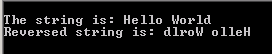Tutorials

# C String Reverse

In this section, you will study how to reverse a string. You can see in the given example, a recursive function reverse(int i) is created.

In this section, you will study how to reverse a string. You can see in the given example, a recursive function reverse(int i) is created.

# C String Reverse

In this section, you will study how to reverse a string. You can see in the given example, a recursive function reverse(int i) is created. Inside the method, we are calculating the length of a specified string using a library function strlen(). Then string st[i] and st[strlen(st)-i-1] are swapped as: c= st[i];
st[i]=st[strlen(st)-i-1];
st[strlen(st)-i-1]=c;

Statements shown above will reverse the string.

Here is the code:

StringReverse.C

 ``` #include  #include  #include  int reverse(int i); char st[]="Hello World"; void main() {   printf("\nThe string is: %s", st);   reverse(0);   printf("\nReversed string is: %s", st);   getch(); } int reverse(int i) {   if (i<(strlen(st)/2)) {   char c;   c= st[i];   st[i]=st[strlen(st)-i-1];   st[strlen(st)-i-1]=c;   }   return 0; }```

Output will be displayed as:

STRING~1.EXE